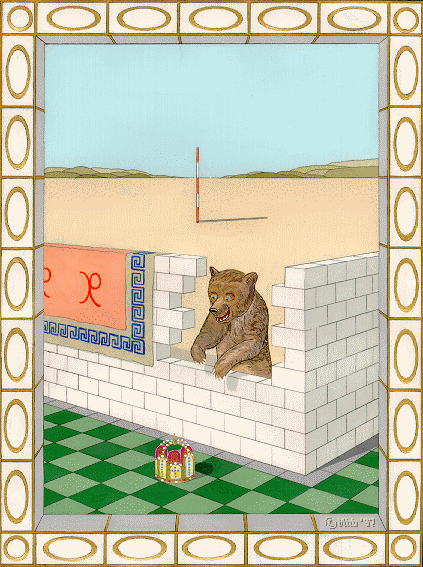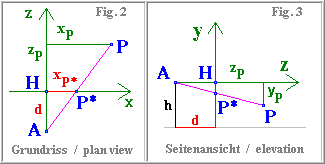Welcome to the perspective page
(designed for MSIE, requires JavaScript, best view 1024 x 768, auxiliary window needed)
Let
S be the ground plane (desert, floor tiles) and B an image plane (the computer screen) perpendicular to S. The observer's eye A is at height h above S and distance d in front of B. The observer is looking straight at B, so we have the principal ray AH, orthogonal to B, which intersects B at the principal point H (see Fig.1).Now imagine a real scene (e. g. the wall) built up on S. Let P be a point in the scene (e.g. the top of the corner of the wall). The position of P is given by three coordinates (xP|yP|zP) in an orthogonal x,y,z coordinate system: The origin is at H, the x and y axes lie in the plane B with the x axis pointing to the right and the y axis upward, while the z axis points away from A to H. The ray AP runs from A to P. Where it intersects B is the image point P* of P; in the x,y coordinate system of the image plane B it has coordinates P*(xP*|yP*).

Calculating the image coordinates P*(xP*| yP*)From the plan view (Fig. 2) we have, by similar triangles, xP* = xP. d / (d + zP),
Similarly, from the side elevation (Fig. 3), we have
yP* = yP. d / (d + zP).

These are our
mapping equations.

Measurements in the image plane (with explanation of terms)
By inverting our mapping equations we can now very easily determine the proportions of various distances in the real scene, knowing where they map to in the image.

How
*) the inset works:
Each cursor position corresponds to a particular image point P* derived from a real point P (see above).
Line 2 shows the coordinates of P* in pixels (origin of coordinates is the principal point H).
Line 3 shows the coordinates of P in metres (origin of coordinates is the eye point A).
Line 5. Radio switch up: if you click on a point in the image, the corresponding real point V becomes the reference point for measuring a length.
Line 6. Radio switch down: the observer's eye A is the reference point.
Line 7 shows the real coordinates of the reference point in metres.
Line 8 shows the distance in metres from the reference point to the real point represented by the cursor.
(Hint: use the numerical arrow keys to move the cursor.)
*) The calculation window can be moved by drag and drop (blue title bar) to a convenient part of the screen.
If coordinates are displayed incorrectly, click the principal point on the horizon 12 pixels away from the right edge of the levelling staff.
If no auxiliary window has opened, allow your browser to do so, or click here: 'no popup'-version

English translation by Hugh Casement;  last update: May. 12. 2011;  main page;   more paintings with animals